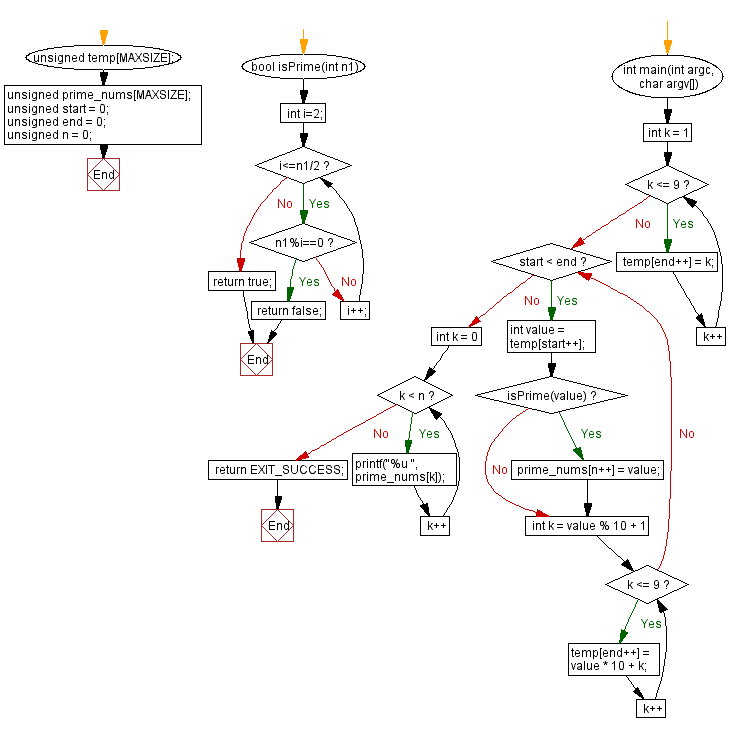﻿ C Program - Prime number in strictly ascending decimal digit order# C Exercises: Prime number in strictly ascending decimal digit order

## C Programming Challenges: Exercise-30 with Solution

Write a C program to create and display all prime numbers in strictly ascending decimal digit order.

Sample Data: 2, 3, 5, 7, 13, 17, 19, 23, 29, 37, 47, 59, 67, 79,..

C Code:

``````#include <stdio.h>
#include <stdlib.h>
#include <stdbool.h>
#include <math.h>
#define MAXSIZE 1000
unsigned temp[MAXSIZE];
unsigned prime_nums[MAXSIZE];
unsigned start = 0;
unsigned end = 0;
unsigned n = 0;
bool isPrime(int n1)
{
int i=2;
while(i<=n1/2)
{
if(n1%i==0)
return false;
else
i++;
}
return true;
}
int main(int argc, char argv[])
{
for (int k = 1; k <= 9; k++)
{
temp[end++] = k;
}

while (start < end)
{
int value = temp[start++];
if (isPrime(value))
{
prime_nums[n++] = value;
}
for (int k = value % 10 + 1; k <= 9; k++)
{
temp[end++] = value * 10 + k;
}
}
for (int k = 0; k < n; k++)
{
printf("%u ", prime_nums[k]);
}

return EXIT_SUCCESS;
}
``````

Sample Output:

```1 2 3 5 7 13 17 19 23 29 37 47 59 67 79 89 127 137 139 149 157 167 179 239 257 2
69 347 349 359 367 379 389 457 467 479 569 1237 1249 1259 1279 1289 1367 1459 14
89 1567 1579 1789 2347 2357 2389 2459 2467 2579 2689 2789 3457 3467 3469 4567 46
79 4789 5689 12347 12379 12457 12479 12569 12589 12689 13457 13469 13567 13679 1
3789 15679 23459 23567 23689 23789 25679 34589 34679 123457 123479 124567 124679
125789 134789 145679 234589 235679 235789 245789 345679 345689 1234789 1235789
1245689 1456789 12356789 23456789
```

Flowchart:## C Programming Code Editor:

Contribute your code and comments through Disqus.

Previous C Programming Exercise: Minimum number of characters to make a palindrome.
Next C Programming Exercise: Arithmetic and geometric progression series.

What is the difficulty level of this exercise?

Test your Programming skills with w3resource's quiz.

﻿